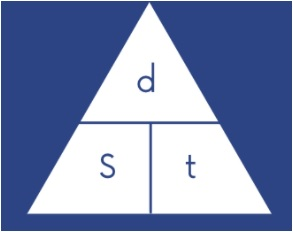Select Page

## Can you confidently rearrange one of the most commonly used physics equations?

### What is Speed?

Speed is a measure of how fast an object is going. This is a concept you need to understand at KS3 and KS4 Physics. There are many keywords you need to have a good understanding of, linked to speed, these include acceleration, braking, deceleration, stationary and distance travelled. Make sure you know what each word means and that you can use it correctly when describing an object’s speed and movement.

### Equations

There are many equations you need to learn in physics, one of the most commonly used is Speed = Distance / Time. This equation is essential in science and maths, you will be expected to know it and be able to use it confidently in both subjects. It is worth remembering that you will also not always be calculating the speed, you may be given that but asked to work out the time taken to travel a certain distance whilst moving at a set speed. Or you could be asked to calculate the distance an object has travelled in a set time whilst moving at a speed of 20mph (for example). This means you need to rearrange the speed equation so you must have the formulae triangle learnt and be able to write it out correctly. This way you can then recall any of the three equations at the drop of a hat. We recommend you practise writing these regularly, in different ways without looking at your notes from a textbook just to check you have learnt and remembered them. Start by drawing out the formulae triangle, if you have learnt this the rest should come easily.### Units

When calculating speed you must always include the units the speed will be measured in. There are three units commonly used, mph (miles per hour), m/s (metres per second) and km/h (kilometres per hour). Which unit you use depends on how the time and distance has been measured. Often you will be given the units but not always, so you look for which units have already been used.

### Distance – Time Graphs

In the topic of speed, we use Distance-Time graphs to show how far an object has travelled in a set period of time. The graph can be split into sections and you are expected to be able to know what each section tells you. Also the direction the line is in will give you more information. You may need to be able to explain the journey an object e.g. person, car or bicycle is taking from interpreting the graph. The graph can also be used to calculate the speed, by reading the measurement of distance and time at a set point. You can then input these values into the equation for speed and calculate the answer.

Hopefully, this gives you a peek into the Speed topic and the key ideas you need to be learning and practising when it comes to revision for upcoming tests. If there are any other topics you would like to us to share our tips and ideas on then make sure you send us an email on kelly@inmyelement-uk.com

Don’t forget to get in touch if you have any questions or would like some help with your KS3 science learning to fill in any knowledge gaps you have.